## 贴片电阻

R值
~

### 检索结果

* 请点击上方检索按钮，查看结果。

57028

### 型号编码规则

1. 1
RC
名称
2. 2
S
功能
3. 3
1608
尺寸(mm)
4. 4
J
公差
5. 5
472
电阻值
6. 6
CS
封装
1. 1RC1608J472CS

#### 系列代码

• RC = 标准
• RH = 不含铅
• RU = 厚膜 CSR
• RJ = 宽端子 CSR
• RL = 金属 CSR
• RF = 扁平阵列
• RP = 凸阵列
• RN = 凹阵列
• RM = 反向凹阵列
• RK = 半反向凹阵列
2. 2RCS1608J472CS

#### 功能代码

• ( ) = 分立
• T = 顶部安装
• B = 反向安装
• K = 低电阻温度系数
• Z = 跳线
• S = 抗硫化
• A = 汽车
• W = 高功率
• P = 金属电镀
3. 3RCS1608J472CS

#### 尺寸- 公制

• 0603 = 0.6 X 0.3 mm
• 1005 = 1.0 X 0.5 mm
• 1608 = 1.6 X 0.8 mm
• 2012 = 2.0 X 1.2 mm
• 3216 = 3.2 X 1.6 mm
• 3225 = 3.2 X 2.5 mm
• 5025 = 5.0 X 2.5 mm
• 6432 = 6.4 X 3.2 mm
• 102P = 1.0 X 0.5 (2片)
• 104P = 1.0 X 0.5 (4片)
• 162P = 1.6 X 0.8 (2片)
• 164P = 1.6 X 0.8 (4片)
4. 4RCS1608J472CS

#### 容量公差代码

• F = A ± 1.0 % E-96 系列
• G = A ± 2.0 % E-48 系列
• J = A ± 5.0 % E-24 系列
• K = A ± 10.0 % E-12 系列
• M = A ± 20.0 % E-6 系列
5. 5RCS1608J472CS

#### 电阻代码

左边2个或3个的数字代表电阻值/ 右边第一个数字代表是10的指数

示例) 472 = 47 X 102 = 4.7㏀ / 1373 = 137 X 103 = 137㏀
1R0 = 1 X R(小数点) X 100 = 1.0Ω (000才是jumper; 1R0是1奥姆,不是jumper)

6. 6RCS1608J472CS

#### 封装代码

• CS = 7 英寸卷盘
• ES = 10 英寸卷盘
• AS = 13 英寸卷盘

### 生产状况说明

• · Mass Production : 批量生产中
• · Not Preferred : 不推荐用于新品开发
• · To Be Discontinued : 购买前请先咨询
• · Discontinued : 已停产

### 电阻器类型说明

ASR 极佳的抗硫化特点，普通和阵列 长效可靠的电子设备、服务器系统(内存模块/硬盘驱动器)、网络设备
Array 二元和四元贴片电阻器 起到阻尼作用、上拉/下拉、数字电路端子、内存模块
CSR 极低电阻值, 电流感测, 厚膜, 金属板 CPU, DC-DC转换器、逆变器、移动通讯设备、电池管理系统（BMS）的电流感测(current sensing)
Typical 普通型、厚膜 智能手机, PC, 数字电视, 相机, 液晶显示器, 内存模块,游戏机, 直流-直流转换器

### 结构说明

Standard 分立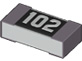Array 凹阵列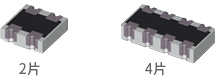ASR 抗硫化分立式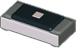CSR 低电阻分立式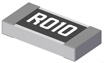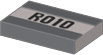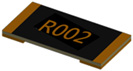High Power 分立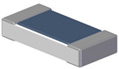### 用途说明

Use for Description data

Automotive 车用, 厚膜电阻
General 普通型, 厚膜电阻
High Power 高电力, 高电压，抗突波, 厚膜电阻
Pb-free 无铅, 厚膜电阻